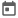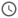# Constraints in System Verilog (Part – 2)##### 21 Oct 2023##### 7 mins

In this article, we will continue our discussion on constraints in System Verilog, which are a way to specify the conditions or restrictions on the random values generated by randomization. In the previous article, we covered the basics of constraint, constraint mode, soft and solve-before keywords. Continuing our discussion, we will cover advance topics of constraint which we can use to create different scenarios for our testbench. We will also see some examples of how to use these conditions in our code.

### Conditions in constraint

There are several types of conditions that we can use in our constraints to define the range, or relationship of the random variables. Some of the common conditions are:

• Equality: We can use `==` operator to specify that a random variable should be equal to a certain value or expression. For example, `constraint c1 {a == 10;}` means that variable `a` should be equal to 10.
• Inequality: We can use `!=` operator to specify that a random variable should not be equal to a certain value or expression. For example, `constraint c2 {b != 0;}` means that variable `b` should not be equal to 0.
• Range: We can use `inside` keyword to specify that a random variable should be within a certain range or set of values. For example, `constraint c3 {c inside {[0:9]};}` means that variable `c` should be between 0 and 9 inclusive. We can also use `{}` to specify a list of values. For example, `constraint c4 {d inside {1,3,5,7};}` means that variable `d` should be one of the values 1, 3, 5, or 7.
• Logical operators: We can use logical operators such as `&&`, `||`, and `!` to combine multiple conditions. For example, `constraint c7 {(h == i) || (h == j) && !(i == j);}` means that either variable `h` should be equal to variable `i`, or variable `h` should be equal to variable `j` and variable `i` should not be equal to variable `j`.
We must not confuse `==` with `=` i.e., assignment operator. In constraint when we use equality operator it is very common to confuse it with assignment operator which is not valid in constraints, as in constraint we can only provide conditions and not assignment.

### Implication operator

Implication operator can be thought of as a simplified version of an if-else snippet. We can use `->` operator to specify that a condition should imply another condition. This means that the condition mentioned in the RHS of implication operator will be applied only when the condition on LHS is true.

Implication operator is different from if-else, as there is no one else part in implication operator, thus a condition is either applied, or it is not applied. This is useful as in most cases we would not want a condition to be applied if the first condition is not true.

#### Example

``````class packet;
typedef enum {DATA, ACK, NACK} pkt_type_e;
rand pkt_type_e type;
rand bit [7:0] length;
constraint c1 {
type inside {DATA, ACK, NACK};
(type == DATA) -> length inside {[1:255]};
}
endclass``````

The constraint `c1` applies the following conditions:

• The `type` variable can be any of the three enum values: DATA, ACK, or NACK.
• If the `type` variable is DATA, then the `length` variable can be any value from 1 to 255.
• If the `type` variable is not DATA, then the implication constraint does not apply. However, this does not mean that the `length` variable are unconstrained. They may still be constrained by other constraints in the class or object.

### Distribution Constraints

Distribution constraints are constraints that specify how likely a variable is to take a certain value or range of values. They are useful for creating scenarios that follow a certain probability distribution or pattern.

Often, we would want to constraint some scenarios to happen more frequently and some scenarios to happen less frequently. This can easily be controlled using a distribution operator. For example, let us suppose we are generating random error packets for our test, but we want the error packets to be generated only 20% of the time. We can use the distribution operator such that out of 100 packets only 20 packets will be erroneous.

We can use two operators to specify the distribution of a variable: `:=` and `:/`. The `:=` operator assigns a fixed weight to a value or range of values, while the `:/` operator assigns a relative weight to a value or range of values.

#### Difference between := and :/

Let us understand the difference using below snippets:

``<var> dist { [100:102]:=2, 200 := 4 };``

For above expression, weights of 100, 101 and 102 will be 2 and for 200 it will be 4. Total of the weights = 2 + 2 + 2 + 4 = 10. So, 100 will be generated (2/10)/100 = 20% times. Similarly, 200 will be generated 40% of the time.

``<var> dist { [100:102] :/ 2, 200 :/4 };``

For above distribution weight of 2 will be distributed amongst 100, 101 and 102, thus each having weight of 2/3. Total weight in this case = 2 + 4 = 6. So 100 will be generated (2/12) / 100 = 16.6% times. Whereas 200 will be generated (4/6)*100 = 66.67% times.

Thus, we can see that the distribution completely changes depending on the operator we are using. But the results will be the same if we do not apply the operator on a range. For example, `dist {100:/2, 300 :/ 2};` and `dist {100 := 2, 300 := 2};` will yield same result.

Distribution operator does not work with randc variables.

#### Example

``````class packet;
rand bit [7:0] length;
constraint c1 {
length dist {
[64:192] := 80,
[0:63]   :/ 10,
[193:255]:/ 10
};
}
endclass``````

The constraint `c1` applies the following conditions:

• The `length` variable can be any value from 0 to 255.
• The `length` variable has an 80% chance of being in the range [64:192], which is the mean plus or minus standard deviation.
• The `length` variable has a 10% chance of being in the range [0:63], which is the lower tail of the distribution.
• The `length` variable has a 10% chance of being in the range [193:255], which is the upper tail of the distribution.

### Randomizing arrays

In SV, we can randomize both static, dynamic arrays, and queues as well. Thus, there needs to be a way to constrain the elements and size in case of dynamic array.

Arrays cannot be constrained directly and thus we need to loop through all the elements of the array and then we can provide constrain on individual elements. The simplest way to iterate through all the elements of array is foreach loop.

#### Example

``````rand bit[7:0] data [];
constraint data_size() {
data.size() inside [4:6];

}
constraint data_c {
foreach(data[i]) {
data[i] < 20;
}
}``````

The constraint `data_size` will limit the size of the dynamic array `data` between 4 to 6.

The constraint `data_c` will constraint the elements of the array to take value less than 20.

### Other features of constraint

In system Verilog, constraints are treated just like a method. Thus, we have all the properties of methods applicable to constraints as well.

#### Constraint Inheritance

Just like any task/function declared inside a class, we can inherit the constraints declared inside the parent class in child class. The constraints can also be over-ridden in the child class like any other method.

`randomize` task is a virtual task and thus it will automatically use the constraints of child class if any of the constraints are overridden.

#### Static Constraint

Constraints can also be declared as static known as static constraints. For static constraints if we call `constraint_mode` to enable/disable constraints, then it will affect all instances of specified constraint in all objects.

In the case of non-static constraints `constraint_mode`only affects the object on which it has been called.

### Example: Packet Generator

Suppose we have a packet generator that can generate packets with different fields: header (8 bits), payload (variable length). The header field contains the packet type (2 bits) and the payload length (6 bits). The packet type determines the format of the payload as follows:

00Data
01Command
10Response
otherInvalid
``````class packet;
rand bit [1:0] pkt_type;
rand bit [5:0] length;
rand bit [7:0] checksum;

//constraint for pkt_type
constraint pkt_type_c {
pkt_type dist { [0:2] :/ 90, 3 :/ 10 };
}

// header should be concatenation of pkt_type and length if pkt_type
// is not 3
(pkt_type != 3) -> header == {pkt_type, length};
}

//constraint for length
constraint length_c {
solve pkt_type before length;
(pkt_type == 0 || pkt_type == 3) -> length inside {[1:4], 8, 16};
(pkt_type == 1 || pkt_type == 2) -> length == 1;
}

// payload size should be equal to length
// payload values should depend on pkt_type
// If pkt_type is Data
if (pkt_type == 2'b00) {
}
// If pkt_type is Command
else if (pkt_type == 2'b01) {
}
// If pkt_type is Response
else if (pkt_type == 2'b10) {
}
// If pkt_type is Invalid
else {
// payload values should be zero
}
}
}

function void post_randomize();
\$display("Packet after randomization:");
endfunction
endclass

module test;
packet pkt;
initial begin
pkt = new;
repeat(10) begin
pkt.randomize();
end
end
endmodule``````
Try this code in EDA Playground
##### Output
``````# Packet after randomization:
# pkt_type = 1, length = 1, header = 41, payload = '{247}
#
# Packet after randomization:
# pkt_type = 2, length = 1, header = 81, payload = '{65}
#
# Packet after randomization:
# pkt_type = 2, length = 1, header = 81, payload = '{143}
#
# Packet after randomization:
# pkt_type = 0, length = 2, header = 02, payload = '{21, 25}
#
# Packet after randomization:
# pkt_type = 0, length = 1, header = 01, payload = '{78}
#
# Packet after randomization:
# pkt_type = 3, length = 3, header = 61, payload = '{0, 0, 0}
#
# Packet after randomization:
# pkt_type = 0, length = 8, header = 08, payload = '{65, 34, 125, 0, 39, 97, 34, 87}
#
# Packet after randomization:
# pkt_type = 0, length = 16, header = 10, payload = '{87, 53, 114, 62, 54, 7, 93, 16, 57, 105, 71, 64, 31, 7, 122, 71}
#
# Packet after randomization:
# pkt_type = 0, length = 3, header = 03, payload = '{108, 52, 53}
#
# Packet after randomization:
# pkt_type = 2, length = 1, header = 81, payload = '{173}
``````

### Conclusion

In this article, we have learned about the concept and usage of constraints in System Verilog, which are a powerful feature to control the randomization of variables and objects. We have seen how to use several types of conditions, implications, and distributions to specify the desired range or relationship of the random values. We have also learned how to randomize arrays and queues, and how to use constraints on their elements and size. Finally, we have applied our knowledge to create a packet generator that can generate several types of packets with various fields.

System Verilog provides diverse operators and flexibility in defining the constraints for variables which ultimately helps in writing TB with highly randomized and controlled stimulus. Thus, we can achieve cover corner cases which might be missed otherwise.